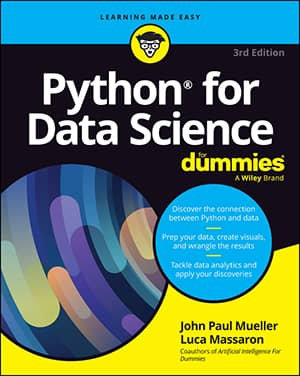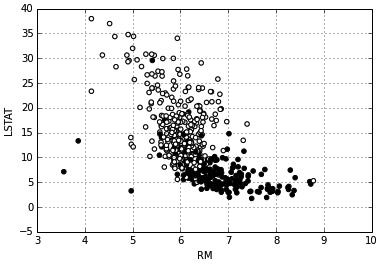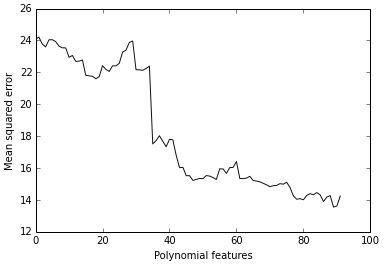##### Python for Data Science For DummiesData scientists can use Python to create interactions between variables. In a linear combination, the model reacts to how a variable changes in an independent way with respect to changes in the other variables. In statistics, this kind of model is a main effects model.

The Naïve Bayes classifier makes a similar assumption for probabilities, and it also works well with complex text problems.

Even though machine learning works by using approximations and a set of independent variables can make your predictions work well in most situations, sometimes you may miss an important part of the picture. You can easily catch this problem by depicting the variation in your target associated with the conjoint variation of two or more variables in two simple and straightforward ways:

• Existing domain knowledge of the problem: For instance, in the car market, having a noisy engine is a nuisance in a city car but considered a plus for sports cars (everyone wants to hear that you got an ultra-cool and expensive car). By knowing a consumer preference, you can model a noise level variable and a car type variable together to obtain exact predictions using a predictive analytic model that guesses the car’s value based on its features.

• Testing combinations of different variables: By performing group tests, you can see the effect that certain variables have on your target variable. Therefore, even without knowing about noisy engines and sports cars, you could have caught a different average of preference level when analyzing your dataset split by type of cars and noise level.

The following example shows how to test and detect interactions in the Boston dataset. The first task is to load a few helper classes:

```from sklearn.linear_model import LinearRegression
from sklearn.cross_validation import cross_val_score
from sklearn.cross_validation import KFold
regression = LinearRegression(normalize=True)
crossvalidation = KFold(n=X.shape, n_folds=10, shuffle=True, random_state=1)```

The code reinitializes the pandas DataFrame using only the predictor variables. A for loop matches the different predictors and creates a new variable containing each interaction. The mathematical formulation of an interaction is simply a multiplication.

```df = pd.DataFrame(X,columns=boston.feature_names)
baseline = np.mean(cross_val_score(regression, df, y, scoring=‘r2’, cv=crossvalidation,
n_jobs=1))
interactions = list()
for feature_A in boston.feature_names:
for feature_B in boston.feature_names:
if feature_A > feature_B:
df[‘interaction’] = df[feature_A] * df[feature_B]
score = np.mean(cross_val_score(regression, df, y, scoring=‘r2’,
cv=crossvalidation, n_jobs=1))
if score > baseline:
interactions.append((feature_A, feature_B, round(score,3)))
print ‘Baseline R2: %.3f’ % baseline
print ‘Top 10 interactions: %s’ % sorted(interactions, key=lambda(x):x,
reverse=True)[:10]
Baseline R2: 0.699
Top 10 interactions: [(‘RM’, ‘LSTAT’, 0.782), (‘TAX’, ‘RM’, 0.766),<br/> (‘RM’, ‘RAD’, 0.759), (‘RM’, ‘PTRATIO’, 0.75), <br/>(‘RM’, ‘INDUS’, 0.748), (‘RM’, ‘NOX’, 0.733), <br/>(‘RM’, ‘B’, 0.731), (‘RM’, ‘AGE’, 0.727), <br/>(‘RM’, ‘DIS’, 0.722), (‘ZN’, ‘RM’, 0.716)]```

The code tests the specific addition of each interaction to the model using a 10 folds cross-validation. It records the change in the R2 measure into a stack (a simple list) that an application can order and explore later.

The baseline R2 is 0.699, so a reported improvement of the stack of interactions to 0.782 looks quite impressive! It’s important to know how this improvement is made possible. The two variables involved are RM (the average number of rooms) and LSTAT (the percentage of lower-status population).

```colors = [‘k’ if v > np.mean(y) else ‘w’ for v in y]
scatter = df.plot(kind=‘scatter’, x=‘RM’, y=‘LSTAT’, c=colors)```

This scatterplot clarifies the improvement. In a portion of houses at the center of the plot, it’s necessary to know both LSTAT and RM in order to correctly separate the high-value houses from the low-value houses; therefore, an interaction is indispensable in this case.Combined variables LSTAT and RM help to separate high from low prices.

Adding interactions and transformed variables leads to an extended linear regression model, a polynomial regression. Data scientists rely on testing and experimenting to validate an approach to solving a problem, so the following code slightly modifies the previous code to redefine the set of predictors using interactions and quadratic terms by squaring the variables:

```polyX = pd.DataFrame(X,columns=boston.feature_names)
baseline = np.mean(cross_val_score(regression, polyX, y, <br/>scoring=‘mean_squared_error’,
cv=crossvalidation, n_jobs=1))
improvements = [baseline]
for feature_A in boston.feature_names:
polyX[feature_A+’^2’] = polyX[feature_A]**2
improvements.append(np.mean(cross_val_score(regression, polyX, y,
scoring=‘mean_squared_error’, cv=crossvalidation, n_jobs=1)))
for feature_B in boston.feature_names:
if feature_A > feature_B:
polyX[feature_A+’*’+feature_B] = polyX[feature_A] * polyX[feature_B]
improvements.append(np.mean(cross_val_score(regression, polyX, y,
scoring=‘mean_squared_error’, cv=crossvalidation, n_jobs=1)))```

To track improvements as the code adds new, complex terms, the example places values in the improvements list. Here is a graph of the results that demonstrates some additions are great because the squared error decreases, and other additions are terrible because they increase the error instead.Adding polynomial features increases the predictive power.

Of course, you could perform an ongoing test to add a quadratic term or interaction optionally, which is called a univariate and greedy approach. This example is a good foundation for checking other ways of controlling the existing complexity of your datasets or the complexity that you have to induce with transformation and feature creation in the course of data exploration efforts. Before moving on, you check both the shape of the actual dataset and its cross-validated mean squared error.

```print shape(polyX)
crossvalidation = KFold(n=X.shape, n_folds=10, shuffle=True, random_state=1)
print ‘Mean squared error %.3f’ % abs(np.mean(cross_val_score(regression, <br/>polyX, y,
scoring=‘mean_squared_error’, cv=crossvalidation, n_jobs=1)))
(506, 104)
Mean squared error 13.466```

Even though the mean squared error is good, the ratio between 506 observations and 104 features isn’t good at all.

As a rule of thumb, there should be 10–20 observations for every coefficient you want to estimate in linear models. However, experience shows that having at least 30 of them is better.